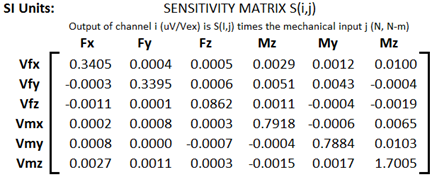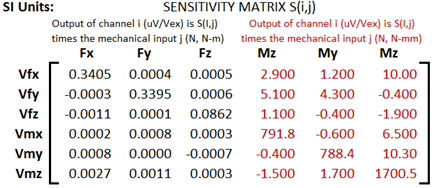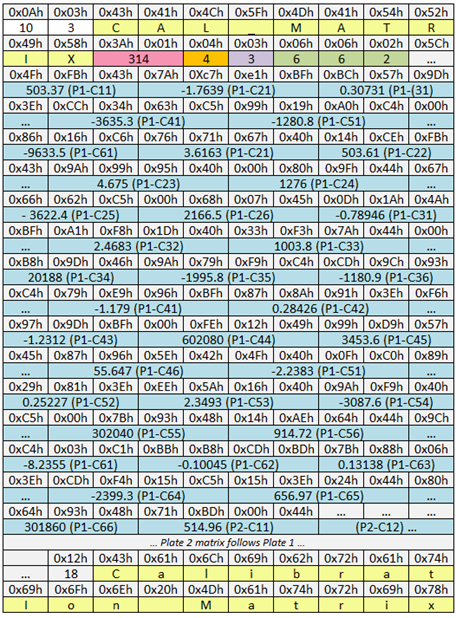﻿ FORCE_PLATFORM:CAL_MATRIX

### FORCE_PLATFORM:CAL_MATRIX

A calibration matrix enables software applications to correct for cross talk between outputs of the force platform; software applications that use the full calibration matrix to correct for cross talk will typically provide more accurate results when compared to applications that only have access to the major diagonal component.Figure 43 - A typical manufacturer’s crosstalk matrix in supplied in Newton-meters

Since the CAL_MATRIX parameter will be ignored, even if present, unless the force platform type is a supported TYPE, its inclusion in a C3D file does not automatically imply that it must be applied to the stored force data.  If the force data TYPE does not support the CAL_MATRIX then the force plate’s data must be scaled using the ANALOG:SCALE factors as described in detail in the chapter entitled Calculating SCALE values for force plates.

Note that most force plate systems include some degree of variable amplification of the signals from the plate.  The amount of amplification applied to each force signal must be taken into account when applying the calibration matrix and is an important factor is the calculation of the correct ANALOG:SCALE value for each force plate channel.

The calibration matrix for each force platform must be applied to the measured channel outputs to obtain the corrected channel outputs according to the matrix equation:

[CAL_MATRIX] Fmeasured = Fcorrected

where the F’s are column vectors.  The elements of the calibration matrix will always be stored in column order i.e., for the first force platform using a 6x6 CAL_MATRIX:

CAL_MATRIX(1,1,1) must contain the first element of the matrix.

CAL_MATRIX(6,1,1) the last element of the first column.

CAL_MATRIX(1,2,1) must contain the first element of the second column, etc.

The first three rows of the supplied calibration matrix have units of force/Volt (e.g.  N/V) and the last three rows have units of moments/Volt (e.g.  N•m/V).  If the C3D file is using distance units of millimeters then the last three rows of the calibration matrix must have units of N•mm/V.  In order to convert from N•m/V to N•mm/V each element in the last three rows must be multiplied by 1000.Figure 44 - The crosstalk matrix with forces scaled in N-m and moments scaled in N-mm.

Note that the analog channels associated with force platforms using the CAL_MATRIX must be scaled in Volts – see the earlier discussions for full details on calculating the analog scale values for each force platform type.  Sample data files and spreadsheets are available from the C3D web site that implements the CAL_MATRIX parameter calculations for the associated analog channels.

When implementing the CAL_MATRIX parameter it is very important to be aware of the order in which the C3D format stores the elements of the matrix, the storage sequence is in column order (as in FORTRAN) and not row order (as in C and C++).  Also, every C3D file uses a consistent set of units throughout – thus while the force plate manufacturer usually supplies the moment calibration data in terms of N•m/V, the calibration matrix must store the moment data in N•mm/V if the POINT calibration and measurement units are millimeters.Figure 45 - A hex dump of a CAL_MATRIX parameter

For example, if we have a 6x6 CAL_MATRIX parameter stored in the C3D file then the first three rows will have units of newtons per Volt and the second three rows will have units of newton•millimeters per Volt (Nm/V * 1000):

C11 C12 C13 C14 C15 C16

C21 C22 C23 C24 C25 C26

C31 C32 C33 C34 C35 C36

C41 C42 C43 C44 C45 C46

C51 C52 C53 C54 C55 C56

C61 C62 C63 C64 C65 C66

If the analog signals from the six force plate sensors are scaled as Volts in the column vector V

V1

V2

V3

V4

V5

V6

Resulting in the corrected forces and moments as the column vector W

W1

W2

W3

W4

W5

W6

Then using the standard notation

W = C*V

Note that W1 is computed by

W1 = C11*V1 +C12*V2 +C13*V3 +C14*V4 +C15*V5 +C16*V6

And that the resulting W1,W2,W3 will have units of newtons, and W4,W5,W6 will have units of newton•millimeters.

The presence of the FORCE_PLATFORM:CAL_MATRIX parameter in a C3D file means that users and researchers retain the ability to determine the quality of the force plate data in a C3D file in any environment instead of trusting that unseen calculations were performed correctly in the past.